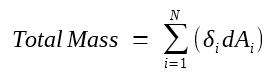# Surface Integral

Surface integral is the area integral of a scalar function or vector field over a specified surface.

This is best understood by looking at a practical problem shown below.

#### The Problem:

The area density (the mass per unit area) of this surface varies with x and y, we need to find the total mass of the surface.#### The Solution:

We can divide the surface into two-dimensional segments over each of which the area density δ(x,y) is approximately constant. For each segment, the density is δi, the area is dAi, and the mass for this small segment is δi dAi.

Then the total mass will be the sum of the mass of all segments:The smaller we make the individual segments, the closer this gets to the true mass of the whole surface.

When we make the dAi approach zero and the number of segments N approach infinity, this sum becomes an surface integration:This the area integral of the scalar function δ(x,y) over the surface S. It is simply a way of adding up the contributions of little pieces of a function (the density in this case) to find a total quantity.